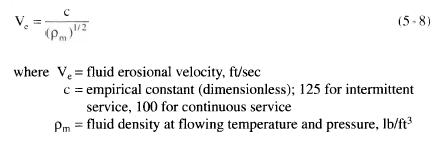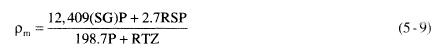﻿ LTX Coil Sizing – Choose Coil Diameter | Oil & Gas Process Engineering

## LTX Coil Sizing – Choose Coil Diameter

Erosional flow criteria will almost always govern in choosing the diameter. Sometimes it is necessary to
check for pressure drop in the coil. Typically, pressure drop will not be important since the whole purpose of the line heater is to allow a large pressure drop that must be taken. The allowable erosional velocity is given by:The fluid density must be for the combined stream of oil and gas and should be calculated at the average gas temperature.where (SG) = specific gravity of liquid relative to water
P = operating pressure, psia
R = gas/liquid ratio, ft3/bbl
S = specific gravity of gas at standard conditions
T = operating temperature, °R
Z = gas compressibility factor

The required pipe internal diameter can be calculated based on the volumetric flow rate and a maximum velocity. The maximum velocity may be the erosional velocity or a limiting value based on noise or inability to use corrosion inhibitors. In gas lines it is recommended that the maximum allowable velocity would be 60 ft/sec, 50 ft/sec if CO2 is present, or the erosional velocity, whichever is lower. (Please note that API Spec 12 K Indirect Type Oil Field Heaters uses 80 ft/sec as a limit.) In liquid lines a maximum velocity of 15 ft/sec should be used. A minimum velocity of 3 ft/sec should also be considered to keep liquids moving and to keep sand or other solids from settling and becoming a plugging problem. The equation used for determining the pipe diameter is:where d = pipe inside diameter, in.
Z = gas compressibility factor
R = gas/liquid ratio, ft3/bbl
T = operating temperature, °R
P = pressure, psia
Q1 = liquid flow rate, bbl/day
V = maximum allowable velocity, ft/sec